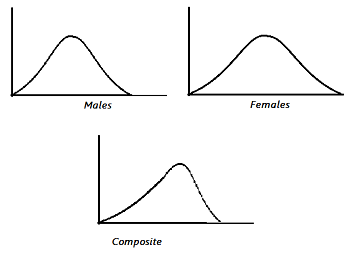# Implicit Factors: Definition, Examples

Statistics Definitions > Implicit Factors

## What are Implicit Factors?

Implicit factors imply some connection between items in a sample. If your sample has a lack of independence, that’s an indication that there may be one or more implicit factors in your sample. The word “factor” is extremely broad, and it means that practically anything can be an implicit factor. Implicit factors can be difficult to detect; to pinpoint the factors affecting your sample, you really need to be familiar with your data and your data collection methods.

## Time as a Factor

One common implicit factor is time. Values collected over periods of time (i.e. every minute, every hour or every year) can be serially correlated. For example, you might be running an experiment to see how diet affects weight gain in teenagers. A person’s weight can increase over time due to normal human growth, which means that time and weight gain are correlated. Time effects can sometimes be found by making an index plot of the data value vs. row number.

## Effects on Experimental Outcomes

Implicit factors can affect the outcome of your experiment in ways you didn’t expect. Let’s say you’re working with data for people’s weights. You find that both males and females follow a normal distribution. However, when you merge the data, you end up with a non normal distribution.This distribution may be skewed, like the composite distribution in the above image, or it might have a non normal kurtosis, both of which will affect any probabilities you calculate from the distribution.

The easy answer to assessing your data for normality would be to use a test for normality to detect any underlying problems. However, if your sample size is too small, your test may not have enough power to detect problems (hence the importance of being familiar with your data).

CITE THIS AS:
Stephanie Glen. "Implicit Factors: Definition, Examples" From StatisticsHowTo.com: Elementary Statistics for the rest of us! https://www.statisticshowto.com/implicit-factors/